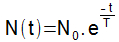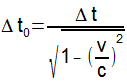Modern Physics

# Time dilation

Time dilation proposes that the time interval marked by a stationary observer is greater than that marked by someone in motion.

Before the proposals made by Albert Einstein about movements with speeds close to that of light , time was treated as something absolute, that is, the passage of time should occur in the same way everywhere and for all possible observers.

The Theory of Relativity , proposed by Einstein at the beginning of the 20th century, showed that time is relative and maintains an interdependent relationship with space. According to this theory, two observers who have relative motion to each other mark different time intervals when observing the occurrence of two successive phenomena.

In theory, relativity proposes time dilation . The time intervals marked by an observer at rest are always longer than the time intervals marked by an observer moving with the speed of light.

Evidence

The existence of the subatomic particles muons evidences the veracity of time dilation. Muons are particles that form from the contact of cosmic rays coming from the Sun with the Earth’s atmosphere. The average lifetime of these particles, before they decay and transform into other elements, is only 2.2 μs (read microseconds. 1μs is 1 million times smaller than 1 second). The decay of muons obeys the following statistical law.• N(t): number of muons that have decayed as a function of time;
• 0 : initial amount of particles;
• t: time;
• T: mean lifetime of muons.

Through Classical Mechanics , considering the idea of ​​average velocity and the above equation, we can define the amount of these particles detected in the soil. Knowing that the particles propagate with a speed that corresponds to 99% of the speed of light and considering a distance of 15 km from layers of the atmosphere , the muon decision time would be approximately 50 μs, and the amount of muons that would arrive to the ground before decaying would be approximately 130 for each trillion particles initially formed.

Experiments carried out to detect muons show that the amount of particles that reach the ground is approximately four trillion eight hundred billion. As these particles have a speed close to the speed of light, the decision time for the muon frame is shorter than the time determined by an observer fixed on the ground. If the decision time relative to the muon frame is used, the theoretical prediction of the amount of particles that reach the ground will be exactly equal to the amount detected experimentally.

Time dilation equation

The following equation determines the relationship between the time intervals marked by an observer at rest and another in motion.The elements of this equation are:

• Δt 0 = Time interval marked by the stationary observer;
• Δt = Time interval marked by the moving observer;
• v = Velocity of the moving body;
• c = Speed ​​of light ( c = 3.0 x 10 8 m/s ).

Through this equation, it can be seen that the time interval marked by the stationary observer is always greater than that marked by the moving observer.